# Introduction to XGBoost in Python

By Ishan Shah and compiled by Rekhit Pachanekar

Ah! XGBoost! The supposed miracle worker which is the weapon of choice for machine learning enthusiasts and competition winners alike. It is said that XGBoost was developed to increase computational speed and optimize model performance.

As we were tinkering with the features and parameters of XGBoost, we decided to build a portfolio of five companies and applied XGBoost model on it to create a trading strategy. Here’s what we got. The five companies were Apple, Amazon, Netflix, Nvidia and Microsoft.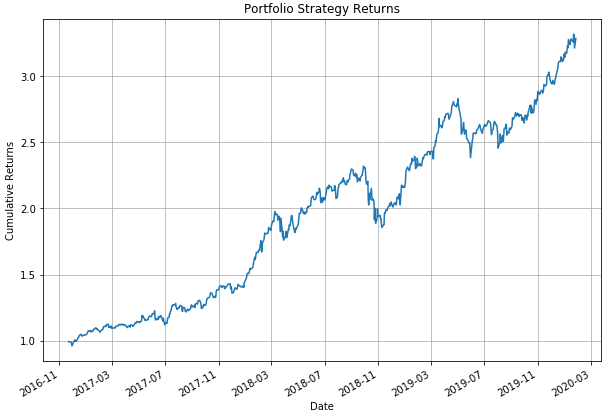That’s really decent. And to think we haven’t even tried to optimise it.

Let’s figure out how to implement the XGBoost model in this article. We will cover the following things:

Before proceeding, if you wish to get into the basics of creating your own Python trading bot and want to delve into the exciting world of algorithmic trading, then you should definitely check out this video. It comprehensively explores the ins and outs of algorithmic trading using Python, catering to both beginners and experienced traders alike.

Let's proceed with XGBOoost!

## What is XGBoost?

Xgboost stands for eXtreme Gradient Boosting and is developed on the framework of gradient boosting. I like the sound of that, Extreme! Sounds more like a supercar than an ML model, actually.

But that is exactly what it does, boosts the performance of a regular gradient boosting model.

“XGBoost used a more regularized model formalization to control over-fitting, which gives it better performance”.

• Tianqi Chen, the author of XGBoost

Let’s break down the name to understand what XGBoost does.

### What is boosting?

The sequential ensemble methods, also known as “boosting”, creates a sequence of models that attempt to correct the mistakes of the models before them in the sequence. The first model is built on training data, the second model improves the first model, the third model improves the second, and so on.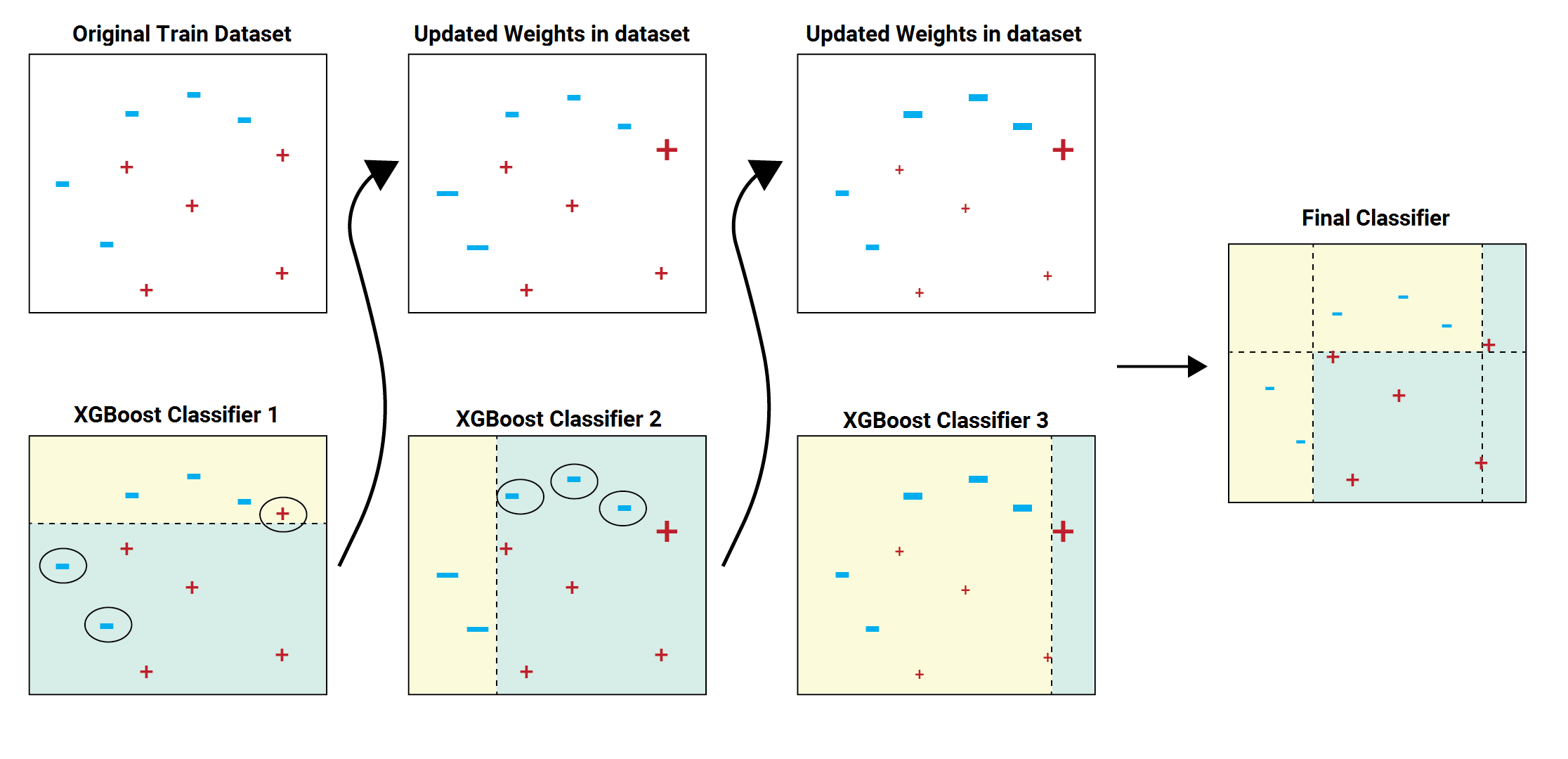In the above image example, the train dataset is passed to the classifier 1. The yellow background indicates that the classifier predicted hyphen and blue background indicates that it predicted plus. The classifier 1 model incorrectly predicts two hyphens and one plus. These are highlighted with a circle. The weights of these incorrectly predicted data points are increased and sent to the next classifier. That is to classifier 2. The classifier 2 correctly predicts the two hyphen which classifier 1 was not able to. But classifier 2 also makes some other errors. This process continues and we have a combined final classifier which predicts all the data points correctly.

The classifier models can be added until all the items in the training dataset is predicted correctly or a maximum number of classifier models are added. The optimal maximum number of classifier models to train can be determined using hyperparameter tuning.

Take a pause over here. Maybe you don’t know what a sequential model is. Let’s take baby steps here.

### Machine learning in a nutshell

Earlier, we used to code a certain logic and then give the input to the computer program. The program would use the logic, ie the algorithm and provide an output. All this was great and all, but as our understanding increased, so did our programs, until we realised that for certain problem statements, there were far too many parameters to program.

And then some smart individual said that we should just give the computer (machine) both the problem and the solution for a sample set and then let the machine learn.

While developing the algorithms for machine learning, we realised that we could roughly put machine learning problems in two data sets, classification and regression. In simple terms, classification problem can be that given a photo of an animal, we try to classify it as a dog or a cat (or some other animal). In contrast, if we have to predict the temperature of a city, it would be a regression problem as the temperature can be said to have continuous values such as 40 degrees, 40.1 degrees and so on.

Great! We then moved on to decision tree models, Bayesian, clustering models and the like. All this was fine until we reached another roadblock, the prediction rate for certain problem statements was dismal when we used only one model. Apart from that, for decision trees, we realised that we had to live with bias, variance as well as noise in the models. This led to another bright idea, how about we combine models, I mean, two heads are better than one, right? This was and is called Ensemble learning. But here, we can use much more than one model to create an ensemble. Gradient boosting was one such method of ensemble learning. .

In gradient boosting while combining the model, the loss function is minimized using gradient descent. Technically speaking, a loss function can be said as an error, ie the difference between the predicted value and the actual value. Of course, the less the error, the better is the machine learning model.

Gradient boosting is an approach where new models are created that predict the residuals or errors of prior models and then added together to make the final prediction.

The objective of the XGBoost model is given as:

Obj = L + Ω

Where L is the loss function which controls the predictive power, and Ω is regularization component which controls simplicity and overfitting

The loss function (L) which needs to be optimized can be Root Mean Squared Error for regression, Logloss for binary classification, or mlogloss for multi-class classification.

The regularization component (Ω) is dependent on the number of leaves and the prediction score assigned to the leaves in the tree ensemble model.

It is called gradient boosting because it uses a gradient descent algorithm to minimize the loss when adding new models. The Gradient boosting algorithm supports both regression and classification predictive modelling problems.

All right, we have understood how machine learning evolved from simple models to a combination of models. Somehow, humans cannot be satisfied for long, and as problem statements became more complex and the data set larger, we realised that we should go one step further. This leads us to XGBoost.

## Why is XGBoost so good?

XGBoost was written in C++, which when you think about it, is really quick when it comes to the computation time. The great thing about XGBoost is that it can easily be imported in python and thanks to the sklearn wrapper, we can use the same parameter names which are used in python packages as well.

While the actual logic is somewhat lengthy to explain, one of the main things about xgboost is that it has been able to parallelise the tree building component of the boosting algorithm. This leads to a dramatic gain in terms of processing time as we can use more cores of a CPU or even go on and utilise cloud computing as well.

While machine learning algorithms have support for tuning and can work with external programs, XGBoost has built-in parameters for regularisation and cross-validation to make sure both bias and variance is kept at a minimal. The advantage of in-built parameters is that it leads to faster implementation.

Let’s discuss one such instance in the next section.

## Xgboost feature importance

Features, in a nutshell, are the variables we are using to predict the target variable. Sometimes, we are not satisfied with just knowing how good our machine learning model is. I would like to know which feature has more predictive power. . There are various reasons why knowing feature importance can help us. Let us list down a few below:

• If I know that a certain feature is more important than others, I would put more attention to it and try to see if I can improve my model further.
• After I have run the model, I will see if dropping a few features improves my model.
• Initially, if the dataset is small, the time taken to run a model is not a significant factor while we are designing a system. But if the strategy is complex and requires a large dataset to run, then the computing resources and the time taken to run the model becomes an important factor.

The good thing about XGBoost is that it contains an inbuilt function to compute the feature importance and we don’t have to worry about coding it in the model.

The sample code which is used later in the XGBoost python code section is given below:

from xgboost import plot_importance
# Plot feature importance
plot_importance(model)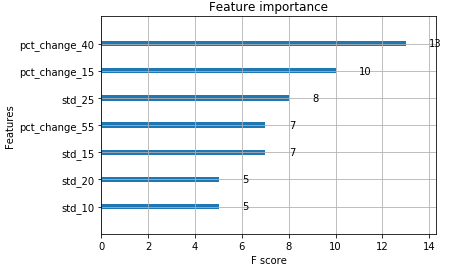All right, before we move on to the code, let’s make sure we all have XGBoost on our system.

## How to install XGBoost in anaconda?

Anaconda is a python environment which makes it really simple for us to write python code and takes care of any nitty-gritty associated with the code. Hence, I am specifying the step to install XGBoost in Anaconda. It’s actually just one line of code.

You can simply open the Anaconda prompt and input the following: pip install XGBoost

The Anaconda environment will download the required setup file and install it for you. It would look something like below.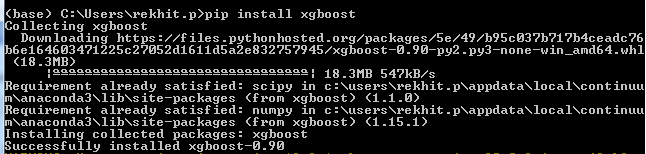That’s all there is to it. Awesome! Now we move to the real thing, ie the XGBoost python code.

## Xgboost in Python

Let me give a summary of the XGBoost machine learning model before we dive into it. We are using the stock data of tech stocks in the US such as Apple, Amazon, Netflix, Nvidia and Microsoft for the last sixteen years and train the XGBoost model to predict if the next day’s returns are positive or negative.

We have included the code in the form of a downloadable python notebook for you to work on later. It is attached at the end of the blog.

We will divide the XGBoost python code into following sections for a better understanding of the model

1. Import libraries
2. Define parameters
3. Creating predictors and target variables
4. Split the data into train and test
5. Initialising the XGBoost machine learning model
6. Cross Validation in Train dataset
7. Train the model
8. Feature Importance
9. Prediction Report

### Import libraries

We have written the use of the library in the comments. For example, since we use XGBoost python library, we will import the same and write # Import XGBoost as a comment.

# Import warnings and add a filter to ignore them
import warnings
warnings.simplefilter('ignore')
# Import XGBoost
import xgboost
# XGBoost Classifier
from xgboost import XGBClassifier
# Classification report and confusion matrix
from sklearn.metrics import classification_report
from sklearn.metrics import confusion_matrix
# Cross validation
from sklearn.model_selection import KFold
from sklearn.model_selection import cross_val_score
# Pandas datareader to get the data
# To plot the graphs
import matplotlib.pyplot as plt
import seaborn as sn
# For data manipulation
import pandas as pd
import numpy as np

Great! All libraries imported. Now we move to the next section.

### Define parameters

We have defined the list of stock, start date and the end date which we will be working with in this blog.

# Set the stock list
stock_list = ['AAPL', 'AMZN', 'NFLX', 'NVDA','MSFT']
# Set the start date and the end date
start_date = '2004-1-1'
end_date = '2020-1-28'

Just to make things interesting, we will use the XGBoost python model on companies such as Apple, Amazon, Netflix, Nvidia and Microsoft. Creating predictors and target variables

We define a list of predictors from which the model will pick the best predictors. Here, we have the percentage change and the standard deviation with different time periods as the predictor variables.

The target variable is the next day's return. If the next day’s return is positive we label it as 1 and if it is negative then we label it as -1. You can also try to create the target variables with three labels such as 1, 0 and -1 for long, no position and short.

Let’s see the code now.

# Create a placeholder to store the stock data
stock_data_dictionary = {}
for stock_name in stock_list:
# Get the data
df = data.get_data_yahoo(stock_name, start_date, end_date)
# Calculate the daily percent change
# create the predictors
predictor_list = []
for r in range(10, 60, 5):
df['pct_change_'+str(r)] = df.daily_pct_change.rolling(r).sum()
df['std_'+str(r)] = df.daily_pct_change.rolling(r).std()
predictor_list.append('pct_change_'+str(r))
predictor_list.append('std_'+str(r))
# Target Variable
df['return_next_day'] = df.daily_pct_change.shift(-1)
df['actual_signal'] = np.where(df.return_next_day > 0, 1, -1)
df = df.dropna()
# Add the data to dictionary
stock_data_dictionary.update({stock_name: df})

Before we move on to the implementation of the XGBoost python model, let’s first plot the daily returns of Apple stored in the dictionary to see if everything is working fine.

# Set the figure size
plt.figure(figsize=(10, 7))
# Access the dataframe of AAPL from the dictionary
# and then compute and plot the returns
(stock_data_dictionary['AAPL'].daily_pct_change+1).cumprod().plot()
# Set the title and axis labels and plot grid
plt.title('AAPL Returns')
plt.ylabel('Cumulative Returns')
plt.grid()
plt.show()

You will get the output as follows: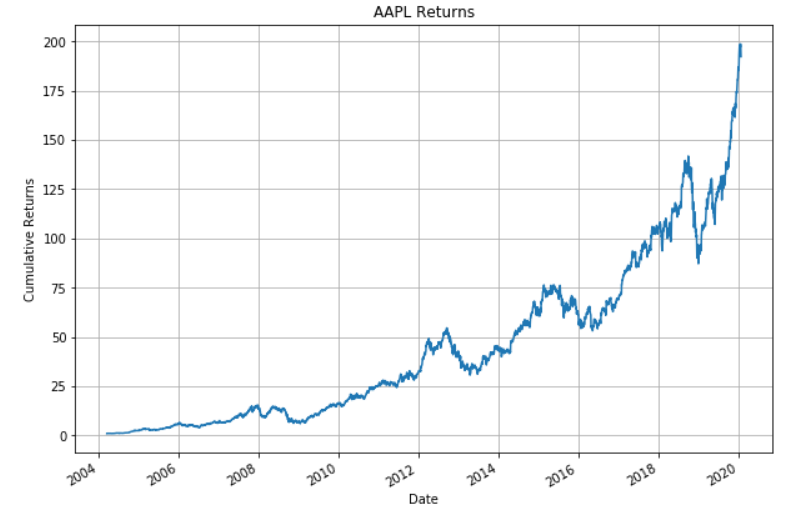It looks accurate.

### Split the data into train and test

Since XGBoost is after all a machine learning model, we will split the data set into test and train set.

# Create a placeholder for the train and test split data
X_train = pd.DataFrame()
X_test = pd.DataFrame()
y_train = pd.Series()
y_test = pd.Series()
for stock_name in stock_list:
# Get predictor variables
X = stock_data_dictionary[stock_name][predictor_list]
# Get the target variable
y = stock_data_dictionary[stock_name].actual_signal
# Divide the dataset into train and test
train_length = int(len(X)*0.80)
X_train = X_train.append(X[:train_length])
X_test = X_test.append(X[train_length:])
y_train = y_train.append(y[:train_length])
y_test = y_test.append(y[train_length:])

### Initialising the XGBoost machine learning model

We will initialize the classifier model. We will set two hyperparameters namely max_depth and n_estimators. These are set on the lower side to reduce overfitting.

# Initialize the model and set the hyperparameter values
model = XGBClassifier(max_depth=2, n_estimators=30)
model

The output is as follows: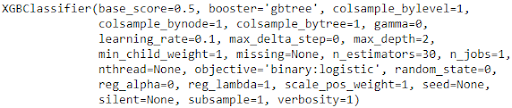All right, we will now perform cross-validation on the train set to check the accuracy.

### Cross Validation in Train dataset

# Initialize the KFold parameters
kfold = KFold(n_splits=5, random_state=7)
# Perform K-Fold Cross Validation
results = cross_val_score(model, X_train, y_train, cv=kfold)
# Print the average results
print("Accuracy: %.2f%% (%.2f%%)" % (results.mean()*100, results.std()*100))

The output is as follows: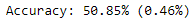The accuracy is slightly above the half mark. This can be further improved by hyperparameter tuning and grouping similar stocks together. I will leave the optimization part on you. Feel free to post a comment if you have any queries.

### Train the model

We will train the XGBoost classifier using the fit method.

# Fit the model

model.fit(X_train, y_train)

You will find the output as follows: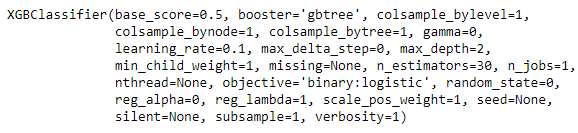### Feature importance

We have plotted the top 7 features and sorted based on its importance.

# Plot the top 7 features
xgboost.plot_importance(model, max_num_features=7)
# Show the plot
plt.show()That’s interesting. The XGBoost python model tells us that the pct_change_40 is the most important feature of the others. Since we had mentioned that we need only 7 features, we received this list. Here’s an interesting idea, why don’t you increase the number and see how the other features stack up, when it comes to their f-score. You can also remove the unimportant features and then retrain the model. Would this increase the model accuracy? I leave that for you to verify.

Anyway, onwards we go!

### Predict and Classification report

# Predict the trading signal on test dataset
y_pred = model.predict(X_test)
# Get the classification report
print(classification_report(y_test, y_pred))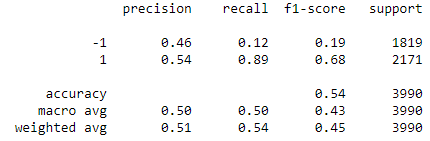Hold on! We are almost there. Let’s see what XGBoost tells us right now:

That’s interesting. The f1-score for the long side is much more powerful compared to the short side. We can modify the model and make it a long-only strategy.

Let’s try another way to formulate how well XGBoost performed.

### Confusion Matrix

array = confusion_matrix(y_test, y_pred)
df = pd.DataFrame(array, index=['Short', 'Long'], columns=[
'Short', 'Long'])
plt.figure(figsize=(5, 4))
sn.heatmap(df, annot=True, cmap='Greens', fmt='g')
plt.xlabel('Predicted')
plt.ylabel('Actual')
plt.show()

The output will be as shown below: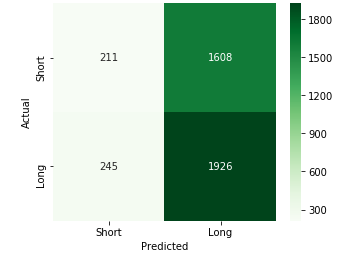But what is this telling us? Well it’s a simple matrix which shows us how many times XGBoost predicted “buy” or “sell” accurately or not. For example, when it comes to predicting “Long”, XGBoost predicted it right 1926 times whereas it was incorrect 1608 times.

Another interpretation is that XGBoost tended to predict “long” more times than “short”.

### Individual stock performance

Let’s see how the XGBoost based strategy returns held up against the normal daily returns ie the buy and hold strategy. We will plot a comparison graph between the strategy returns and the daily returns for all the companies we had mentioned before. The code is as follows:

# Create an empty dataframe to store the strategy returns of individual stocks
portfolio = pd.DataFrame(columns=stock_list)
# For each stock in the stock list, plot the strategy returns and buy and hold returns
for stock_name in stock_list:
# Get the data
df = stock_data_dictionary[stock_name]
# Store the predictor variables in X
X = df[predictor_list]
# Define the train and test dataset
train_length = int(len(X)*0.80)
# Predict the signal and store in predicted signal column
df['predicted_signal'] = model.predict(X)
# Calculate the strategy returns
df['strategy_returns'] = df.return_next_day * df.predicted_signal
# Add the strategy returns to the portfolio dataframe
portfolio[stock_name] = df.strategy_returns[train_length:]
# Plot the stock strategy and buy and hold returns
print(stock_name)
# Set the figure size
plt.figure(figsize=(10, 7))
# Calculate the cumulative strategy returns and plot
(df.strategy_returns[train_length:]+1).cumprod().plot()
# Calculate the cumulative buy and hold strategy returns
(stock_data_dictionary[stock_name][train_length:].daily_pct_change+1).cumprod().plot()
# Set the title, label and grid
plt.title(stock_name + ' Returns')
plt.ylabel('Cumulative Returns')
plt.legend(labels=['Strategy Returns', 'Buy and Hold Returns'])
plt.grid()
plt.show()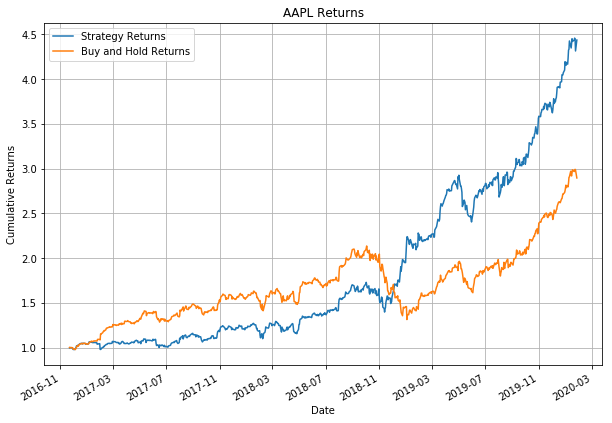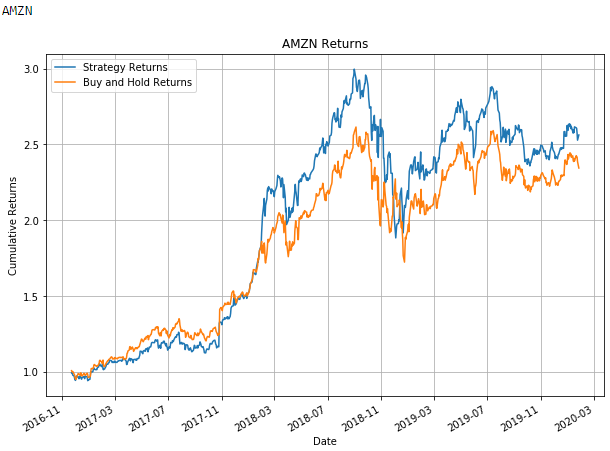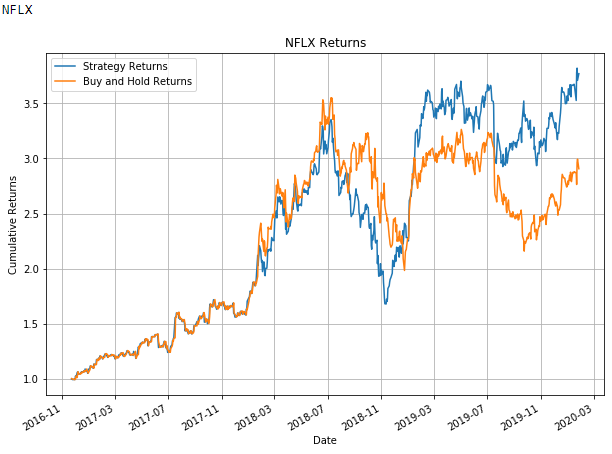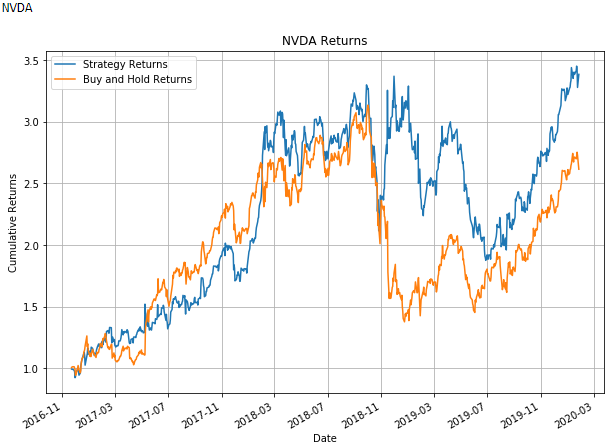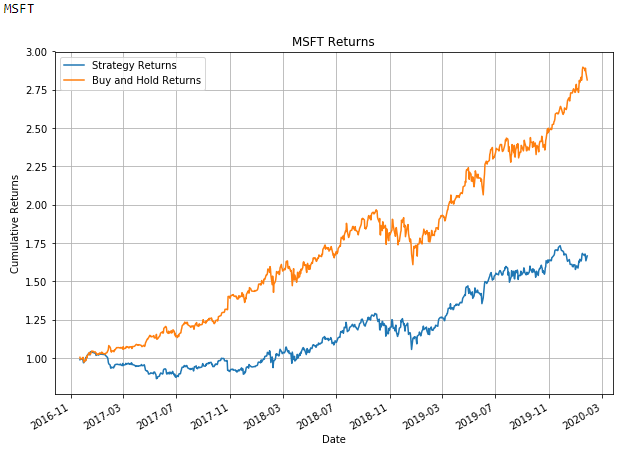This was fun, wasn’t it? What do you think of the comparison? Do let us know your observations or thoughts in the comments and we would be happy to read them.

### Performance of portfolio

We were enjoying this so much that we just couldn’t stop at the individual level. Hence we thought what would happen if we invest in all the companies equally and act according to the XGBoost python model. Let’s see what happens.

# Drop missing values
portfolio.dropna(inplace=True)
# Set the figure size
plt.figure(figsize=(10, 7))
# Calculate the cumulative portfolio returns by assuming equal allocation to the stocks
(portfolio.mean(axis=1)+1).cumprod().plot()
# Set the title and label of the chart
plt.title('Portfolio Strategy Returns')
plt.ylabel('Cumulative Returns')
plt.grid()
plt.show()Well, remember that these are cumulative returns, hence it should give you an idea about the performance of an XGBoost model.

If you want more detailed feedback on the test set, try out the following code.

import pyfolio as pf
pf.create_full_tear_sheet(portfolio.mean(axis=1))

While the output generated is somewhat lengthy, we have attached a snapshot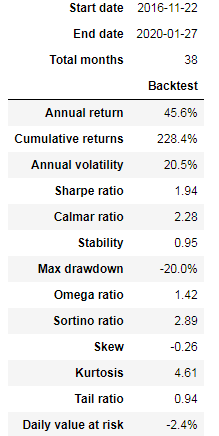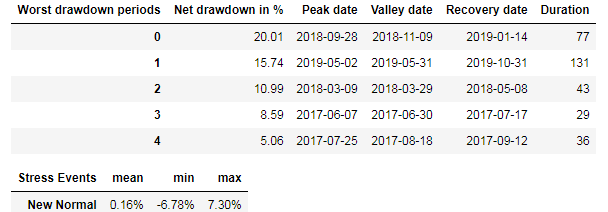Phew! That was a long one. But we hope that you understood how a boosted model like XGBoost can help us in generating signals and creating a trading strategy.

## Conclusion

We started from the base, ie the emergence of machine learning algorithms and its next level, ie ensemble learning. We learnt about boosted trees and how they help us in making better predictions. We finally came to XGBoost machine learning model and how it is better than a regular boosted algorithm. We then went through a simple XGBoost python code and created a portfolio based on the trading signals created by the code. In between, we also listed down feature importance as well as certain parameters included in XGBoost.

If you want to embark on a stepwise training plan on the complete lifecycle of machine learning trading strategies, then you can take the Machine learning strategy development and live trading learning track and receive guidance from experts such as Dr. Ernest P. Chan, Terry Benzschawel and QuantInsti.

Disclaimer: All data and information provided in this article are for informational purposes only. QuantInsti® makes no representations as to accuracy, completeness, currentness, suitability, or validity of any information in this article and will not be liable for any errors, omissions, or delays in this information or any losses, injuries, or damages arising from its display or use. All information is provided on an as-is basis.

Press the Download button to fetch the code we have used in this blog.